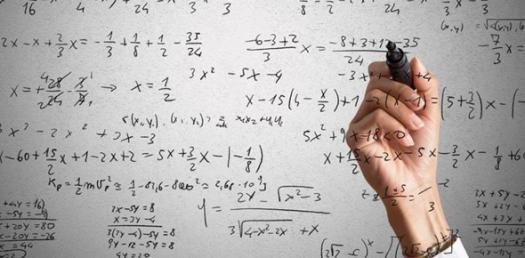# Algebra II Test 1

Approved & Edited by ProProfs Editorial Team
The editorial team at ProProfs Quizzes consists of a select group of subject experts, trivia writers, and quiz masters who have authored over 10,000 quizzes taken by more than 100 million users. This team includes our in-house seasoned quiz moderators and subject matter experts. Our editorial experts, spread across the world, are rigorously trained using our comprehensive guidelines to ensure that you receive the highest quality quizzes.
| By Shelbyo92
S
Shelbyo92
Community Contributor
Quizzes Created: 1 | Total Attempts: 616
Questions: 7 | Attempts: 616SettingsA quiz over the main 8 parent functions. Algebra 2 grade 11

• 1.

### What is the Parent Function for the equation y=x^2?

• A.

Rational

• B.

• C.

Absolute value

Explanation
The parent function for the equation y=x^2 is quadratic. A quadratic function is a polynomial function of degree 2, where the highest power of the variable is 2. In this case, the equation y=x^2 represents a parabola that opens upwards, with the vertex at the origin. The quadratic parent function is the simplest form of a quadratic equation, and all other quadratic equations can be derived from it through transformations.

Rate this question:

• 2.

### The equation for the rational parent function is                      .

y=1/x
Explanation
The equation y=1/x represents the rational parent function. In this equation, the variable y represents the dependent variable and x represents the independent variable. The function describes a hyperbola that passes through the origin (0,0) and has asymptotes at the x-axis and y-axis. As x approaches positive or negative infinity, y approaches zero, and as x approaches zero, y approaches positive or negative infinity. This equation is the standard form of the rational parent function and can be used to graph various rational functions by applying transformations.

Rate this question:

• 3.

### The linear parent function has an equation of...

• A.

Y=2x

• B.

X=3y

• C.

Y=x

C. Y=x
Explanation
The correct answer is y=x because the linear parent function represents a straight line with a slope of 1 and a y-intercept of 0. This means that for every increase of 1 in x, there is a corresponding increase of 1 in y. Therefore, the equation y=x accurately represents the linear parent function.

Rate this question:

• 4.

### The graph for parent function exponential growth movies in an                  direction.

upward
Explanation
The graph for the parent function exponential growth moves in an upward direction because as the input values increase, the output values also increase at an increasing rate. This results in a graph that starts at a low point and steadily rises as the input values increase.

Rate this question:

• 5.

### Y=log(x) is the

• A.

Logarithmic Parent function.

• B.

Absolute Parent function.

• C.

Square root parent function.

A. Logarithmic Parent function.
Explanation
The given equation y=log(x) represents the logarithmic parent function. This is because the equation is in the form of y=log(base)x, which is the general form of a logarithmic function. The logarithmic parent function is the most basic form of a logarithmic function and serves as a reference point for other logarithmic functions. It is characterized by a vertical asymptote at x=0 and a horizontal asymptote at y=0.

Rate this question:

• 6.

### When an equation has 2 bars outside the x it is a

• A.

Exponential growth pf.

• B.

Logarithmic pf.

• C.

Absolute value pf

C. Absolute value pf
Explanation
When an equation has 2 bars outside the x, it indicates that it is an absolute value function. Absolute value functions are represented by two bars enclosing the variable and can be defined as the distance of the variable from zero on a number line, regardless of its sign. Therefore, the correct answer is "absolute value pf".

Rate this question:

• 7.

### When the points equal (0,0), (1,1), (4,2) and (9,3) the pf is..

• A.

Linear. pf

• B.

• C.

Square root. pf

C. Square root. pf
Explanation
The given points form a pattern where the y-coordinate is the square root of the x-coordinate. This indicates that the relationship between the variables is a square root function. Therefore, the correct answer is "Square root. pf".

Rate this question:

Related TopicsBack to top